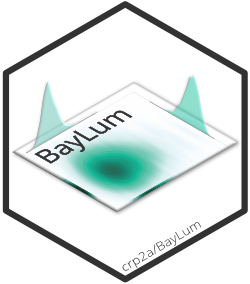## All functions

AgeC14_Computation()

Bayesian analysis for C-14 age estimations of various samples

AgeS

Output of AgeS_Computation function for the samples: "GDB5" and "GDB3"

AgeS_Computation()

Bayesian analysis for OSL age estimation of various samples

Age_Computation()

Bayesian analysis for the OSL age estimation of one sample

Age_OSLC14()

Bayesian analysis for age estimation of OSL measurements and C-14 ages of various samples

BayLum-package BayLum

Chronological Bayesian Models Integrating Optically Stimulated Luminescence and C-14 DatingDATA1

DATA of sample named GDB3

DATA2

DATA on sample named GDB5

DATA3

DATA of sample named FER1

DATA_C14

C14 cal age estiamte and its error

Generate_DataFile()

Generates, from one (or several) BIN-file(s) of Single-grain OSL measurements, a list of luminescence data and information before statistical analysis (DEPRECATED)

Generate_DataFile_MG()

Generates, from one (or several) BIN file(s) of Multi-grain OSL measurements a list of luminescence data and information before statistical analysis (DEPRECATED)

IntCal13

Atmospheric North data for calibration of 14C age

IntCal20

Atmospheric North data for calibration of 14C age

LT_RegenDose()

Plots Lx/Tx as a function of the regenerative dose

MCMCsample

MCMC sample from the posterior distribution of the dataset GDB5

Marine13

Marine data for calibration of 14C age

Marine20

Marine data for calibration of 14C age

ModelC14

Likelihood of C14 samples for JAGS models use in Age_OSLC14

ModelOSL

Likelihood of OSL samples for JAGS models use in Age_OSLC14

ModelPrior

Prior for JAGS models use in Age_OSLC14

Model_Age

JAGS models use in Age_Computation

Model_AgeC14

JAGS models use in AgeC14_Computation

Model_AgeS

JAGS models use in AgeS_Computation

Model_Palaeodose

JAGS models use in Palaeodose_Computation

Palaeodose_Computation()

Bayesian analysis for the palaeodose estimation of various samples

SCMatrix()

Definition of the stratigraphic constraint matrix

SC_Ordered()

Create stratigraphically ordered sample matrix

SHCal13

Atmospheric South data for calibration of 14C age

SHCal20

Atmospheric South data for calibration of 14C age

combine_DataFiles() Concat_DataFile()

Combine objects

create_DataFile()

Prepare input data for subsequent BayLum Analysis

create_FolderTemplates()

Create Folder Templates

create_ThetaMatrix()

Create Theta Matrix

plot_Ages()

Create Age Plot

plot_MCMC()

Plot MCMC trajectories and posterior distributions

plot_Scatterplots() ScatterSamples()

Display Scatter Plot Matrix of the Bayesian Age Results

write_BayLumFiles()

Write BayLum .csv-files

write_YAMLConfigFile()

Write Auto Generated YAML BayLum Configuration File to the Disc